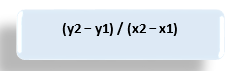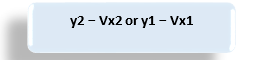# High-Low Method

• Updated on

What is high-low method?

The high-low method is a way to find out the fixed and variable components of a mixed cost. The method collects the cost at a high and a low activity level to extract the fixed and variable cost components. It is a beneficial type of analysis for making pricing and budgeting decisions. Management can use it to determine the fixed and variable parts of costs associated with a product, asset, geography or even a subsidiary. It is a cost accounting technique commonly implied across all types of organisations, across sectors.

Highlights
• High low method segregates the fixed and variable parts of a total cost of a product.
• It helps management and cost accountants ascertain the variable and fixed cost accruing at a per-unit level.
• It is a straightforward method to understand and does not need additional complex tools to compute the costs.

How is the high-low methodology used?There are three types of charges/costs in most businesses: fixed-charge, variable charge, and semi-viable charge or mixed cost. The high low methodology separates the fixed and variable cost components from a mixed charge or a total cost.

It compares the top activity level costs with the bottom level activity costs of operations. It then charts all costs on a graph that connects the top activity costs and lowest activity costs. No complicated tools or programmes are needed to utilise this methodology. It is a very vital tool for cost accountants in determining fixed and variable costs for budgeting purposes. It is also helpful for simulating and predicting the direction of the financials of a company.

What are the formulas employed in high-low method?

The formulas employed in the high-low methodology of forming a cost model has two stages:

First, an estimate of variable cost per unit is done. Then the fixed cost for a certain level of production is decided. Finally, with the steps' results, an approximate cost for the given production level is computed.

We can use a linear equation to compute the total cost to separate the fixed cost (F) and variable cost (V). The equation shall show the cost volume of the total cost.

The function will be-The above y shall be the total cost, and x the production level at which computation is being made.

For this, first, we calculate the variable cost per unit (V), using:Here, y2 is the total cost at the maximum level, and x2 is the number of units at this level.

y1 is the total cost at a minimum level, and x1 is the respective number of units produced.

The slope of this linear equation plotted in a graph will show the change in cost due to the change in production. Then, the total fixed cost can be computed using this. The total variable cost will therefore be deducted from the total cost of production to get it.

So, the formula for fixed cost will be –This formula can be used at the highest and the lowest level of activity to determine fixed costs.

Example of determining fixed cost using high low method-

Doodles International produces 10,000 green panels in June for AU\$50,000 and 5,000 green widgets in July for AU\$35,000. Therefore, there is an addition of AU\$15,000 and 5,000 units in the two periods. Thus, during July, the variable cost per unit will be AU\$15,000 divided by 5,000 units, AU\$3 per unit. From the above calculation, we know that AU\$15,000 of the costs incurred by Doodles in July were variable. Therefore, it means that the remaining AU\$20,000 are fixed costs.

How is the high-low method interpreted?

The high-low method is beneficial for the management in determining how much of a particular product to produce. The fixed costs plus the variable cost per unit can be multiplied by the units produced. It will help obtain the total cost. Reshuffling this formula can help figure out the total fixed costs when unknown. It is easy to understand the relationship between fixed and variable parts of a cost at diverse output levels.

What are the advantages of the high-low method?• The high-low method is the easiest way to separate fixed and variable costs components for a product.
• It only uses easily obtainable values and some algebra to determine cost behaviour.
• It does not use or require cost accountants to employ any complex tools or programmes.
• The method is simple to understand.
• It allows analysts and accountants to forecast unit costs with utter ease.

What are the disadvantages of the High-Low Method?

• The cost-activity relationship taken for this methodology ignores minute details and variations in costs.
• It assumes constant fixed and variable costs per unit, which is not practical.
• Only two data points are considered in the calculation; variations are not captured in the estimate.
• Better estimates and methods for cost computation are available.
• Sometimes the high-low method tends to yield inaccurate results.
• Outlier costs (that are not commonly incurred) are not considered.
• Additional or step costs only incurred at specific volume levels are not taken.
• It provides only an estimate which can be prone to false assumptions.

##### Top ASX Listed Companies

Definition:
We use cookies to ensure that we give you the best experience on our website. If you continue to use this site we will assume that you are happy with it. OK